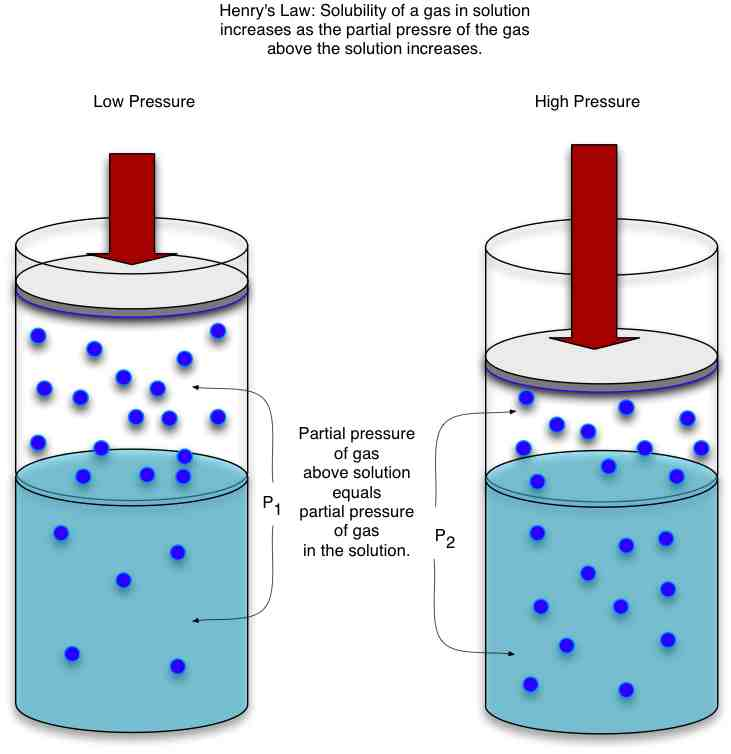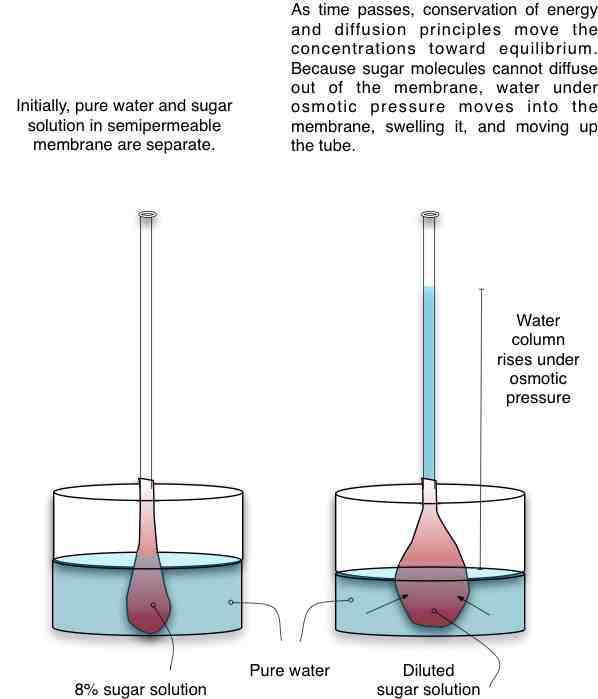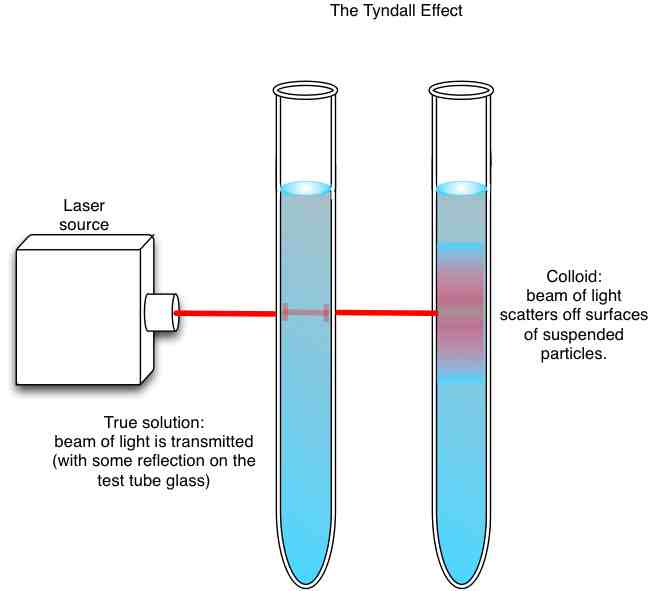# Chemistry

#### Chemistry 13: 3-5WebLecture

# Colligative Properties

## Factors affecting Reaction Rates

Even when two substances can mix together to form a solution, their ability to do so will be affected by pressure and temperature. Solubility is the amount of solute that can dissolve in a solvent. We've already seen that some solids are readily soluble in certain solvents, while others do not readily dissolve at all.

#### Henry's Law

A gas above a liquid will dissolve to some extent in the liquid as gas molecules with enough velocity penetrate the surface tension of the liquid from above, or gain enough velocity to escape the liquid solvent from below. If we increase pressure on the gas above the liquid, more of the gas molecules will be driven into the liquid to dissolve. So the solubility of a gas solute in a liquid is directly proportional to the partial pressure of the gas above the liquid:

Sg = kHPgSome chemists prefer the form Pg = kHcsolute, where c is concentration in moles/liter (Molarity) of the solute. The units and numerical value of kH will change, depending on the form of Henry's law that is used.

In order to determine kH for a given solution, chemists measure the solubility of a gas in solution as the pressure of the gas above the solution is carefully changed. For extremely volatile gases, this determination can be very tricky, so chemists are constantly looking for new experimental methods that will yield more accurate results. To determine Henry's law constants, chemists may use a method called "headspace analysis", in which a solution is heated to a specific temperature in a sealed container. Gases that are volatile (take gas form) above the temperature will evaporate from the solution into the headspace above the liquid surface. This gas is then captured, and its components are separated and analyzed using chomatography and mass spectrometers.

#### Le Chatelier's Principle

When we dissolve either a gas or a solid in a liquid, the solubility depends in a large extent on the temperature of the solvent. Increasing or decreasing temperature, is usually a matter of adding (or withdrawing) energy from the system. A complete description of any chemical reaction must include energy as one of the factors:

reactants → products + energy

Although the reaction arrow points from reactants to products in many equations, the reality is that some molecules are always moving from products to reactant formation, as well as from reactant to product formation. In a product-favored reaction, more products form than reactants. Over time, however, the amount of reactants will decrease and the rate of product formation will drop. When the rate of product formation and the rate of reactant formation are equal, the overall amount of products and reactants stops changing. In a solution, although individual molecules continue to react, the concentrations stop changing. The chemical system is in equilibrium.

In most cases, equilibrium also means that the system is in the lowest and most stable energy state. It requires work to disturb a system in equilibrium, and such a system will attempt to return to an equilibrium state (although this may be a different set of concentrations). We can apply this principle of chemical inertia to solution systems in order to predict which direction a reaction will run in order to re-establish equilibrium if it is disturbed.

Suppose that we consider an equilibrium state where the solution holds as much solute as it can and is saturated. While individual molecules may be leaving or entering the dissolved state, no changes in concentration are occurring. We can write the reaction situation (using a double-headed arrow to show equilibrium) as

solute + solvent ⇔ saturated solution + energy

The reaction can run in either direction, depending on the conditions. If we disturb equilibrium in any way, conservation of energy requires that the system begin a reaction process that tries to neutralize the change. This tendency is called Le Chatelier's Principle, and it allows us to predict what will happen. If we add more solvent to our system above, the reaction will run to the right in order to reduce the amount of solvent present. We will get a new equilibrium state. If we add energy to the system by heating it (increased temperature), the process will run to the left, increasing the amount of solute we can dissolve to create a saturated solution.

This property allows us to create supersaturated solutions — solutions that can hold more than their saturation amount when heated. As a supersaturated solution cools, the solute will slowly precipitate out, forming a solid again. If the solid was ionic to start with, the precipitate will take the form of a growing crystal.### Practice with the Concepts 1

Consider the reaction:

3H2(g) + N2(g) ↔ NH3(g)

When we add NH3 to the reaction, what happens?

## Colligative Properties

We've considered how changes in pressure and temperature affect solubility. These changes do not depend on the kind of molecule involved, but they may depend on the number of molecules involved in a chemical system. Factors such as the boiling point and freezing point of a solution, when they depend only on the ratio of solute particles to solvent particles, are called colligative properties.

#### Raoult's law and Ideal Behavior

We can express the change in behavior between a pure solvent and a solution with Raoult's law:

Ps = XsP°s

Here, Ps is the pressure exerted by the solvent in solution, Xs is the mole fraction of the solvent in solution, and P°s is the pressure the pure solvent would exert. Since X is the ratio of the moles of solvent to the total solution, it is always less than 1, and Ps is always less than P°s.

Raoul's law makes a critical assumption that the molecules of the solute which dilute the solvent properties behave exactly the same way the solvent molecules behave. However, if the solute molecules interact with the solvent molecules, either because of charge, polarity, or size differences, Raoult's law will only give us a proximation of actual behavior.

Henry's Law and Raoult's Law give boundaries for the behavior of a gas.We can also write Raoult's law in terms of the solute:

ΔPsolvent = -XsoluteP°solvent

which is sometimes useful in predicting the amount of change a particular solute dilution level will produce.

#### BP Elevation, FP Suppression

Since changing solution concentrations affects the actual vapor pressure of a substance (solute or solvent) above the liquid phase, concentration changes affect the boiling point, where vapor pressure equals atmospheric pressure. Adding a solute drops vapor pressure, so we must increase temperature for a diluted solution to raise its vapor pressure high enough to make it boil. Diluting a solution raises the boiling point of the solvent. The temperature change is given by

ΔTbp = Kbpmsolute

where Kbp is a constant determined experimentally for each solution, and msolute is the molality of the solute.

Similarly, diluting a solvent with a solute affects the freezing point of the solvent. The solute particles hold the solvent particles apart, making it harder for the solvent to form intermolecular bonds required to change phase to a solid. It is necessary to get the solvent colder to allow these bonds to form. Diluting a solution lowers the freezing point of the solvent. The temperature change is given by:

ΔTfp = Kfpmsolute

Again, Kfp is a constant determined experimentally for each solution. Both Kfp and Kbp have the units of degrees centigrade/molal, °C/m.

It is important to realize that the number of solute particles depends on how the solute dissolves. A molecular substance such as sugar will dissolve into discrete sugar molecules, with the number of particles equal to the molecules of sugar in the pre-dissolved mass. But when an ionic solid dissolves, the anion and cation separate, increasing the number of distinct particles from the solute in the solution. This dissolution is rarely perfect, so the number of solute particles in solution will be some value less than precisely double the ion pairs in the original solid sample. The actual number of solute particles for a given ionic substance must be calculated experimentally, and is called van't Hoff's factor, i. This factor changes the freezing point depression calculation to:

ΔTfp = i * Kfpmsolute

#### Osmotic Pressure

Conservation of energy drives the process of diffusion, by which concentrated solute particles spread out into the solution volume to achieve a uniform distribution. In many biological situations, this diffusion process is blocked by a semipermeable membrane, through which some molecules can pass (usually gases and water) but others (such as sugars) cannot. Instead of the solute particles diffusing into the volumes of lower concentration, water molecules will move into the volumes of higher concentration to equalize concentration levels. When the solvent diffuses instead of the solute, the process is called osmosis.As water moves into the concentrated solution bag, the bag swells and forces the diluting sugar solution up the tube, until the weight of the dilute solution in the tube balances the osmotic pressure driving water through the semipermeable membrane. We can measure the osmotic pressure as a function of the concentration and temperature of the diluted solution:

Π = cRT

#### Colloids

Mixtures are solutions in which the solid particles of solute are so large that they settle out under their own weight. We may call such a mixture a suspension while the particles are partially floating. Rivers carrying sediment are a natural example of this phenomenon, with settlement of the solute particles occurring at the delta.

In a "true" chemical solution, the solute and solvent particles are molecular in size or smaller, and remain permanently in their homogenous state. Solutes in true solutions can diffuse through membranes, and will crystalize in supersaturated solution conditions.

There is a middle range of solutions where the solute particles are too large to diffuse and too irregular to form crystals, but not heavy enough to settle out over time. These solutions are colloids, and they have interesting physical properties that affect their chemical reactivity.

Most of the special properties of colloids come from the very large surface-to-volume ratios of the particles of solute. One phenomenon is the Tyndall effect, in which light is scattered from the surfaces, instead of passing through the solution as it would if the solute particles were on the order of molecular sizes.Colloids also act as surfactants, soaps that can emulsify or surround and attach to substances such as oils, removing them from other surfaces (such as your clothing).

### Practice with the Concepts 2

The freezing point of water is 0 °C, and its freezing point depression constant is 1.86 °C/molal.

How much is the freezing point of water depressed if we add enough sucrose to pure water to attain a sucrose molality of 6 moles/1kg water?

Discussion Questions
• How does the deviation of real materials from ideal situations like those described in Raoult's Law, Henry's Law, and the Ideal Gas Law affect the practice of industrial chemists?